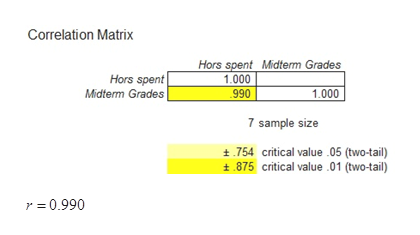# The following data gives the number of hours 7 students spent studying and their corresponding grades on their midterm exams.Hours Spent Studying0.51.52.53.544.55.5Midterm Grades69727884879396 Calculate the correlation coefficient, r. Round your answer to six decimal places.

Question
1 views

The following data gives the number of hours 7 students spent studying and their corresponding grades on their midterm exams.

 Hours Spent Studying Midterm Grades 0.5 1.5 2.5 3.5 4 4.5 5.5 69 72 78 84 87 93 96

Calculate the correlation coefficient, r. Round your answer to six decimal places.

check_circle

Step 1

Step-by-step procedure to obtain the correlation coefficient using Excel-Mega Stat is given below:

• Enter the data into Excel spreadsheet.
• Choose MegaStat > Correlation/Regression > Correlation Matrix.
• In Input ranges, Select the data values as Hours spent and Midterm Grades.
• Click OK.
Step 2

Output obtained using Excel-Mega Stat is represented as follows:

Fro...help_outlineImage TranscriptioncloseCorrelation Matrix Hors spent Midterm Grades 1.000 990 Hors spent Midterm Grades 1.000 7 sample size 754 critical value 05 (two-tail) 875 critical value 01 (two-tail) r = 0.990 fullscreen

### Want to see the full answer?

See Solution

#### Want to see this answer and more?

Solutions are written by subject experts who are available 24/7. Questions are typically answered within 1 hour.*

See Solution
*Response times may vary by subject and question.
Tagged in

### Other